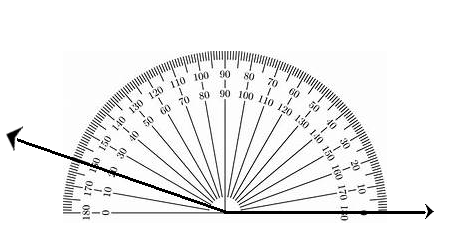# McGraw Hill Math Grade 4 Chapter 13 Lesson 3 Answer Key Drawing Angles

Practice the questions of McGraw Hill Math Grade 4 Answer Key PDF Chapter 13 Lesson 3 Drawing Angles to secure good marks & knowledge in the exams.

## McGraw-Hill Math Grade 4 Answer Key Chapter 13 Lesson 3 Drawing Angles

Draw

Draw an angle with each measure. Use a protractor.

Question 1.
130°Explanation:
The measure of the angle is 130-degrees.

Question 2.
160°Explanation:
The measure of the angle is 160-degrees.

Question 3.
65°Explanation:
The measure of the angle is 65-degrees.

Question 4.
20°Explanation:
The measure of the angle is 20-degrees.

Question 5.
Look at each angle you drew. Classify each as acute, obtuse, or right.
#1 ___________________
#2 ___________________
#3 ___________________
#4 ___________________